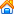网站建设| 数据库类| 图形图象| 程序设计| 现代办公| 操作系统| 考试认证| 网络技术| 软件工程| 电脑相关| 文学作品 网站开发| 网页制作| 操作系统| 图象图形| 考试认证| 数据库类| 程序设计| 硬件技术| 现代办公| 网络技术| 笑话频道
 书籍下载 电脑文摘您的位置： 电脑书库首页-> 电脑文摘-> 网页制作-> HTML-> VBScript教程（三）

VBScript教程（三）

 相关文章： 相关书籍:

・ If...Then...Else 语句
・ Select Case 语句

If...Then...Else 语句用于计算条件是否为 True 或 False，并且根据计算结果指定要运行的语句。通常，条件是使用比较运算符对值或变量进行比较的表达式。有关比较运算符的详细信息，请参阅比较运算符。If...Then...Else 语句可以按照需要进行嵌套。

Sub FixDate()
Dim myDate
myDate = #2/13/95#
If myDate < Now Then myDate = Now
End Sub

If value = 0 Then
End If
End Sub

If value = 0 Then
Else
End If
End Sub

If...Then...Else 语句的一种变形允许您从多个条件中选择，即添加 ElseIf 子句以扩充 If...Then...Else 语句的功能，使您可以控制基于多种可能的程序流程。例如：

Sub ReportValue(value)
If value = 0 Then
MsgBox value
ElseIf value = 1 Then
MsgBox value
ElseIf value = 2 then
Msgbox value
Else
Msgbox "数值超出范围！"
End If

Select Case 结构提供了 If...Then...ElseIf 结构的一个变通形式，可以从多个语句块中选择执行其中的一个。Select Case 语句提供的功能与 If...Then...Else 语句类似，但是可以使代码更加简练易读。

Select Case 结构在其开始处使用一个只计算一次的简单测试表达式。表达式的结果将与结构中每个 Case 的值比较。如果匹配，则执行与该 Case 关联的语句块：

Select Case Document.Form1.CardType.Options(SelectedIndex).Text
Case "MasterCard"
DisplayMCLogo
ValidateMCAccount
Case "Visa"
DisplayVisaLogo
ValidateVisaAccount
Case "American Express"
DisplayAMEXCOLogo
ValidateAMEXCOAccount
Case Else
DisplayUnknownImage
PromptAgain
End Select

・Do...Loop： 当（或直到）条件为 True 时循环。
・While...Wend： 当条件为 True 时循环。
・For...Next： 指定循环次数，使用计数器重复运行语句。
・For Each...Next： 对于集合中的每项或数组中的每个元素，重复执行一组语句。

While 关键字用于检查 Do...Loop 语句中的条件。有两种方式检查条件：在进入循环之前检查条件（如下面的 ChkFirstWhile 示例）；或者在循环至少运行完一次之后检查条件（如下面的 ChkLastWhile 示例）。在 ChkFirstWhile 过程中，如果 myNum 的初始值被设置为 9 而不是 20，则永远不会执行循环体中的语句。在 ChkLastWhile 过程中，循环体中的语句只会执行一次，因为条件在检查时已经为 False。

Sub ChkFirstWhile()
Dim counter, myNum
counter = 0
myNum = 20
Do While myNum > 10
myNum = myNum - 1
counter = counter + 1
Loop
MsgBox "循环重复了 " & counter & " 次。"
End Sub

Sub ChkLastWhile()
Dim counter, myNum
counter = 0
myNum = 9
Do
myNum = myNum - 1
counter = counter + 1
Loop While myNum > 10
MsgBox "循环重复了 " & counter & " 次。"
End Sub

Until 关键字用于检查 Do...Loop 语句中的条件。有两种方式检查条件：在进入循环之前检查条件（如下面的 ChkFirstUntil 示例）；或者在循环至少运行完一次之后检查条件（如下面的 ChkLastUntil 示例）。只要条件为 False，就会进行循环。

Sub ChkFirstUntil()
Dim counter, myNum
counter = 0
myNum = 20
Do Until myNum = 10
myNum = myNum - 1
counter = counter + 1
Loop
MsgBox "循环重复了 " & counter & " 次。"
End Sub

Sub ChkLastUntil()
Dim counter, myNum
counter = 0
myNum = 1
Do
myNum = myNum + 1
counter = counter + 1
Loop Until myNum = 10
MsgBox "循环重复了 " & counter & " 次。"
End Sub

Exit Do 语句用于退出 Do...Loop 循环。因为通常只是在某些特殊情况下要退出循环（例如要避免死循环），所以可在 If...Then...Else 语句的 True 语句块中使用 Exit Do 语句。如果条件为 False，循环将照常运行。

Sub ExitExample()
Dim counter, myNum
counter = 0
myNum = 9
Do Until myNum = 10
myNum = myNum - 1
counter = counter + 1
If myNum < 10 Then Exit Do
Loop
MsgBox "循环重复了 " & counter & " 次。"
End Sub

While...Wend 语句是为那些熟悉其用法的用户提供的。但是由于 While...Wend 缺少灵活性，所以建议最好使用 Do...Loop 语句。

For...Next 语句用于将语句块运行指定的次数。在循环中使用计数器变量，该变量的值随每一次循环增加或减少。

Sub DoMyProc50Times()
Dim x
For x = 1 To 50
MyProc
Next
End Sub

Sub TwosTotal()
Dim j, total
For j = 2 To 10 Step 2
total = total + j
Next
MsgBox "总和为 " & total & "。"
End Sub

Sub NewTotal()
Dim myNum, total
For myNum = 16 To 2 Step -2
total = total + myNum
Next
MsgBox "总和为 " & total & "。"
End Sub

Exit For 语句用于在计数器达到其终止值之前退出 For...Next 语句。因为通常只是在某些特殊情况下（例如在发生错误时）要退出循环，所以可以在 If...Then...Else 语句的 True 语句块中使用 Exit For 语句。如果条件为 False，循环将照常运行。

For Each...Next 循环与 For...Next 循环类似。For Each...Next 不是将语句运行指定的次数，而是对于数组中的每个元素或对象集合中的每一项重复一组语句。这在不知道集合中元素的数目时非常有用。

<HTML>
<SCRIPT LANGUAGE="VBScript">
<!--
Sub cmdChange_OnClick
Dim d '创建一个变量
Set d = CreateObject("Scripting.Dictionary")

For Each I in d
Document.frmForm.Elements(I).Value = D.Item(I)
Next
End Sub
-->
</SCRIPT>
<BODY>
<CENTER>
<FORM NAME="frmForm"

<Input Type = "Text"><p>
<Input Type = "Text"><p>
<Input Type = "Text"><p>
<Input Type = "Text"><p>
<Input Type = "Button" NAME="cmdChange" VALUE="单击此处"><p>
</FORM>
</CENTER>
</BODY>
</HTML>

Sub 过程

Sub 过程是包含在 Sub 和 End Sub 语句之间的一组 VBScript 语句，执行操作但不返回值。Sub 过程可以使用参数（由调用过程传递的常数、变量或表达式）。如果 Sub 过程无任何参数，则 Sub 语句必须包含空括号 ()。

Sub ConvertTemp()
temp = InputBox("请输入华氏温度。", 1)
MsgBox "温度为 " & Celsius(temp) & " 摄氏度。"
End Sub

Function 过程

Function 过程是包含在 Function 和 End Function 语句之间的一组 VBScript 语句。Function 过程与 Sub 过程类似，但是 Function 过程可以返回值。Function 过程可以使用参数（由调用过程传递的常数、变量或表达式）。如果 Function 过程无任何参数，则 Function 语句必须包含空括号 ()。Function 过程通过函数名返回一个值，这个值是在过程的语句中赋给函数名的。Function 返回值的数据类型总是 Variant。

Sub ConvertTemp()
temp = InputBox("请输入华氏温度。", 1)
MsgBox "温度为 " & Celsius(temp) & " 摄氏度。"
End Sub

Function Celsius(fDegrees)
Celsius = (fDegrees - 32) * 5 / 9
End Function

Function Celsius(fDegrees)
Celsius = (fDegrees - 32) * 5 / 9
End Function

Temp = Celsius(fDegrees)

MsgBox "温度为 " & Celsius(fDegrees) & " 摄氏度。"

Call MyProc(firstarg, secondarg)
MyProc firstarg, secondarg[文章录入员:lpc]

 相关文章： 相关书籍: